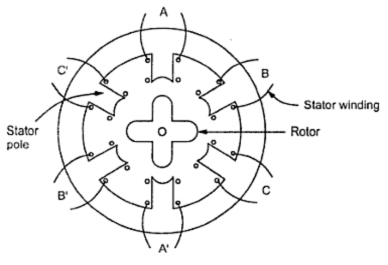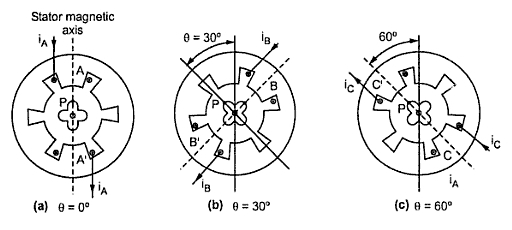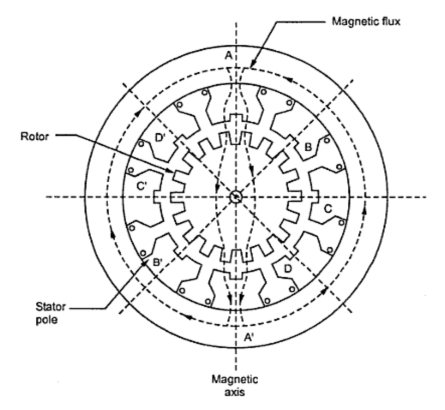### Variable Reluctance Stepper Motors

Stepper Motor Part 2
It is the most basic type of stepper motor. Thus helps to explain the principle of operation of the stepper motors.
The motors has a stator which is usually wound for three phases. The stator has six salient poles with concentrated exciting windings around each one of them. The stator construction is laminated and assembled in a single stack. The number of poles on the stator and rotor are different. This gives th motor ability,
1. of bidirection rotation and
2. self starting capability.
The rotor is made out of slotted steel laminations. If the number of stator poles are Nand the number of rotor poles are Nr then for a three phase motor, the rotor poles interms of and q are given by,

For example for Ns = 6 and q =3, the rotor poles are,
For our discussion, 4 pole rotor construction is selected. So rotor has 4 salient poles without any exciting winding as shown in the Fig.1Fig. 1 Schematic arrangement of variable reluctance motor

The coils wound around diametrically opposite poles are connected in series and the three phases are energized from a d.c. source with the help of switches.
The basic driving circuit is shown in the Fig. 2.Fig. 2  Driving circuit

1.1 Operation
The operation is based on various reluctance positions of rotor with respect to stator. When any one phase of the stator is excited, it produces its magnetic field whose axis lies along the poles, the phase around which is excited. Then rotor moves in such a direction so as to achieve minimum reluctance position. Such a position means a position where axis of magnetic field of stator matches with the axis passing through any two poles of the rotor. Let us see the operation when phases A, B and C are energized in sequence one after the other, with the help of switches SW1, SW2 and SW3.
1. When the phase AA' is excited with the switch SW1 closed, then stator magnetic axis exists along the poles formed due to AA' i.e. vertical. Then rotor adjusts itself in a minimum reluctance position i.e. matching its own axis passing through the two poles exactly with stator magnetic axis. This position is shown in the Fig. 3(a).Fig. 3  Steps in variable reluctance motor

2. When the phase BB' is excited with the switch SW2 closed and phase AA' de-energized with the switch SW1 open, then stator magnetic axis shifts along the poles formed due to BB;, shown dotted in the Fig. 3(b). Then rotor tries to align in the minimum reluctance position and turns through 30o in anticlockwise direction. So axis passing through two diagonally opposite poles of rotor matches with the stator magnetic axis. This is the new minimum reluctance position. The point P shown on the rotor has rotated through 30o in anticlockwise direction as shown in the Fig. 3(b).
3. When the phase CC' is excited with the switch SW3 closed and the phases AA' and BB' are de-energized, then the stator magnetic axis shifts along the poles formed due to CC', shown dotted in the Fig. 3(c). Then to achieve minimum reluctance position, rotor gets subjected to further anticlockwise torque. So it turns through further 30o in anticlockwise direction.
Hence point P is now at 60o from its starting position, in anticlockwise direction as shown in the Fig. 3(c). By successively exciting the three phases in the specific sequecnce, the motor takes twelve steps to complete on revolution.
Now if i is the current passing through the phase which is excited then the torque developed by the motor, which acts on the rotor is expressed as,

where L is the inductance of the relevant phase at an angle θ.
Since the torque is proportional to the torque of the phase current (T  α   i2), it is independent of the direction of i. The direction of rotation is totally decided from the sequence in which the phases are excited.
1.2 Important Observation
From the above discussion, the following important observations can be made :
i) The rotor can be moved in a specific direction, by exciting the stator phases in a specific sequence.
ii) When the phases are excited in the sequence A-B-C-A ...., the rotor moves in the anticlockwise direction, as explained earlier.
iii) When the phases are excited in the sequence C-B-A-C ..., the rotor moves in the clockwise direction, which can be easily verified.
iv) The distance through which the rotor moves when all three phases are excited once is called one rotor tooth pitch.
Rotor tooth pitch = 360o/Nr
v) The step angle is denoted as, and given by,
αs  = 360o/qNr
So for three phases and four rotor poles the step angle is,
αs  = 360o/(3 x 4) = 30o
This is shown in the previous section. If the number of phases are increased to eight and the number of rotor poles to six then the step angle becomes,
αs  360o/(8 x 6) = 7.5o
1.3 Microstepping
In the above discussion we have assumed that the windings are excited one at a time. If the two phases are excited simultaneously i.e. keeping AA' excited, the BB' is also excited with switch SW1 and SW2  closed, then the stator magnetic axis shifts to a mid position rather than along BB'. Hence rotor gets aligned along this moves through a half step i.e. 15o.
A logical extension of this technique is to control the currents in the phase windings so that several stable equilibrium positions are created. Normally the step angle is reduced by factor of 1/2, 1/5, 1/10, 1/16 or 1/32. This technique is called microstepping.
A further reduction in step angle can be achieved by increasing the number of poles of the stator and rotor by adopting different constructions such as,
i) Using reductiongear mechanism.
ii) Using multistack arrangement
1.4 Reduction Gear Stepper Motor
Fig. 4 shows a reduction gear stepper motor. The stator has 8 salient poles and four phases for use as exciting winding. The rotor has 18 teeth and 18 slots uniformly distributed around. Each salient pole of the stator consists of two teeth, forming an interleaving slot of the same angular periphery as the rotor teeth or slots. When the coil A-A' is excited, the resulting electromechanical torque brings the rotor to the position as shown in the Fig. 4.Fig. 4 Reduction gear stepper motor

With this arrangement, the step angle reduces to 5. By successive excitation of coils A-A', B-B', C-C; and D-D', the rotor makes 72 steps to complete one revolution. The general relationship between step angle , number of stator phases q and rotor poles or teeth Nr  remains same as,
αs  = 360o/mNr
By choosing different combinations of number of rotor teeth and stator phases, any desired step angle can be achieved.
1.5 Multistack Stepper Motor
As mentioned earlier, these are used to obtain small step size, typically ranging between 2 to 15o.
In a m stack motor, the motor is divided into a m number of magnetically isolated sections called stacks, along its axial length. The m stacks of stator have a common frame while the rotors are mounted on a common shaft. The stators and rotors have the same number of poles (teeth). The stator poles in all m stacks are aligned while the rotor poles are shifted by (1/m) of the pole pitch from one another. All the stator windings in a stator stack are excited simultaneously hence each stator stack forms a phase. So number of stator phases is equal to number of stator stacks. Generally three stack stepper motors are used. The Fig. f shows the arrangement in three stack stepper motor alongwith shifting of the rotor poles by (1/3) of the pole pitch from one another.Fig. 5 Reduction gear stepper motor

The Fig. 6 shows the cross sectional view of a three stack, three phase variable reluctance motor. In each stack, the stator and rotor laminations have 12 poles. The poles of the stator are in one line while the rotor poles are offset from each other by one third of the pole pitch.Fig. 6 Cross sectional view of 3 stack variable reluctance motor

The various windings in one stack are energized simultaneously. When phase A of stator is excited then rotor poles of stack A get aligned with the stator poles. But due to offset, rotor poles of stack B and C do not align. Now if phase A is de-energized and phase B is energized, rotor poles of stack B get aligned with the stator poles. Thus, rotor moves by one third of pole pitch. When B is de-energized and C excited, rotor further moves by one third of pole pitch so that rotor poles of stack C get aligned with the stator poles.
If m is the number of stacks i.e. phases and N be the rotor poles then the step angle is given by,
αs  = 360o/mNr
In the case discussed above, m = 3 and N = 12 hence the step angle is,
αs  = 360o/(3 x 12) = 10o
An alternative design where the rotor stacks are aligned and stator stacks are offset also is used in practice.
1.6 Advantages of Variable Reluctance Motor
The variable reluctance stepper motor has following advantages.
1. High torque to inertia ratio
2. High rates of acceleration.
3. Fast dynamic response
4. Simple and low cost machine
5. Efficient cooling arrangement as all the windings are on stator and there is no winding on rotor.

#### 1 comment:

1.Great article. I'd like to use variable reluctance stepper motors in a hobby project. Specifically a very simple 4/2 pole configuration. Any tips on where these can be purchased online?